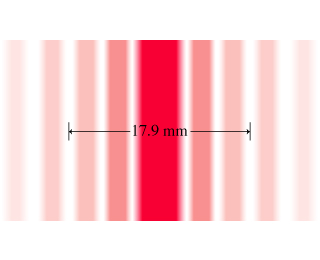# Problem: You have been asked to measure the width of a slit in a piece of paper. You mount the paper 80.0 centimeters from a screen and illuminate it from behind with laser light of wavelength 633 nanometers (in air). You mark two of the intensity minima as shown in the figure, and measure the distance between them to be 17.9millimeters. (Figure 1)Part AWhat is the width, a, of the slit?Express your answer in micrometers, to three significant figures.Part BIf the entire apparatus were submerged in water, would the width of the central peak change?a) The width would increase.b) The width would decrease.c) The width would not change.

###### FREE Expert Solution

Diffraction minima:

Wavelength in water:

$\overline{){{\mathbf{\lambda }}}_{{\mathbf{w}}}{\mathbf{=}}\frac{{\mathbf{\lambda }}_{\mathbf{a}\mathbf{i}\mathbf{r}}}{{\mathbf{\eta }}_{\mathbf{w}}}}$

Part A

For small angles measured in radians;

tan θ ≈ sin θ ≈ opposite/adjacent

opposite = y = (17.9 mm)/2 = 8.95 mm (1m/1000mm) = 8.95 × 10-3 m

adjacent = D = 80.0 cm(1m/100cm) = 0.8 m

λ = 633 nm (10-9m/1nm) = 633 × 10-9 m

90% (210 ratings)###### Problem Details

You have been asked to measure the width of a slit in a piece of paper. You mount the paper 80.0 centimeters from a screen and illuminate it from behind with laser light of wavelength 633 nanometers (in air). You mark two of the intensity minima as shown in the figure, and measure the distance between them to be 17.9millimeters. (Figure 1)Part A

What is the width, a, of the slit?

Part B

If the entire apparatus were submerged in water, would the width of the central peak change?

a) The width would increase.

b) The width would decrease.

c) The width would not change.